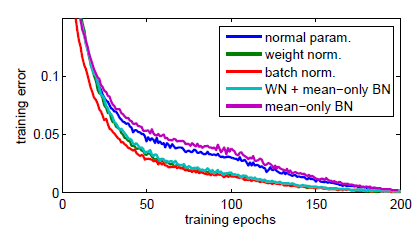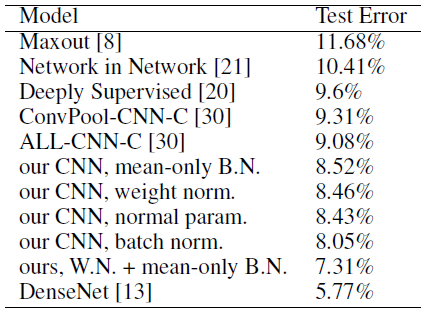# Review — Weight Normalization: A Simple Reparameterization to Accelerate Training of Deep Neural Networks

## Weight Norm, Compared to Batch Norm, Computational Cheaper & Independent of Batch Size

Weight Normalization: A Simple Reparameterization to Accelerate Training of Deep Neural Networks, Weight Norm, by OpenAI,
2016 NIPS, Over 1400 Citations (Sik-Ho Tsang @ Medium)
Image Classification, CNN, Normalization

• Weight normalization, a reparameterization of the weight vectors in a neural network that decouples the length of those weight vectors from their direction.
• It speeds up convergence while it does not introduce any dependencies between the examples in a minibatch. This means that weight norm can also be applied successfully to recurrent models such as LSTMs and to noise-sensitive applications such as deep reinforcement learning.

# Outline

1. Weight Normalization
2. Combining Weight Normalization With Mean-Only Batch Normalization
3. Experimental Results

# 1. Weight Normalization

• In a standard artificial neural network, the computation of each neuron consists in taking a weighted sum of input features x, followed by an elementwise nonlinearity Φ:
• Weight normalization re-parameterizes each weight vector w in terms of a parameter vector v and a scalar parameter g and to perform stochastic gradient descent with respect to those parameters instead.
• The weight vectors are expressed in terms of the new parameters using:
• where v is a k-dimensional vector, g is a scalar, and ||v|| denotes the Euclidean norm of v.
• And we got ||w||=g, which is independent of the parameters v.

By decoupling the norm of the weight vector g from the direction of the weight vector (v/||v||), the convergence of stochastic gradient descent optimization is speed up.

• By differentiating the above equation, the gradients of a loss function L with respect to the new parameters v, g is obtained:

Unlike with batch normalization, the expressions above are independent of the minibatch size and thus cause only minimal computational overhead.

# 2. Combining Weight Normalization With Mean-Only Batch Normalization

• With this normalization method, the minibatch means are subtracted out like with full batch normalization, but it does not divide by the minibatch standard deviations. That is, neuron activations are computed using:
• where w is the weight vector, parameterized using weight normalization, and μ[t] is the minibatch mean of the pre-activation t.
• During training, a running average of the minibatch mean is kept which will be substituted in for μ[t] at test time.

# 3. Experimental ResultsTraining error for CIFAR-10 using different parameterizations

However, training with batch normalization was about 16% slower compared to the standard parameterization. In contrast, weight normalization was not noticeably slower.Classification results on CIFAR-10 without data augmentation

Mean-only batch normalization combined with weight normalization has the best performance at 7.31% test error. It is hypothesized that the noise added by batch normalization can be useful for regularizing the network.

• (There are also experiments on VAE, and deep reinforcement learning in the paper. Please feel free to read if interested.)

[2016 NIPS] [Weight Norm]
Weight Normalization: A Simple Reparameterization to Accelerate Training of Deep Neural Networks

## Image Classification

1989 … 2016 [Weight Norm] … 2021: [Learned Resizer] [Vision Transformer, ViT] [ResNet Strikes Back] [DeiT] [EfficientNetV2] [MLP-Mixer] [T2T-ViT] [Swin Transformer] [CaiT] [ResMLP] [ResNet-RS] [NFNet] [PVT, PVTv1] [CvT] [HaloNet] [TNT] [CoAtNet] [Focal Transformer] [TResNet]

--

--